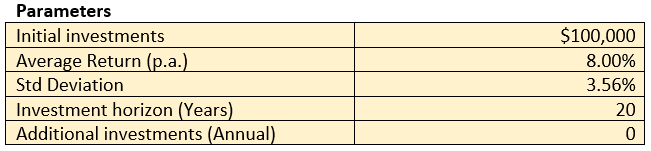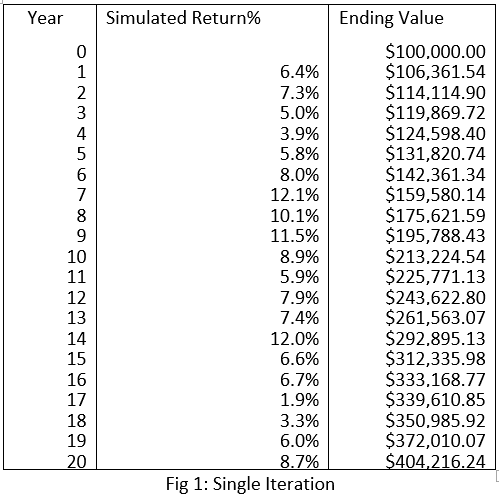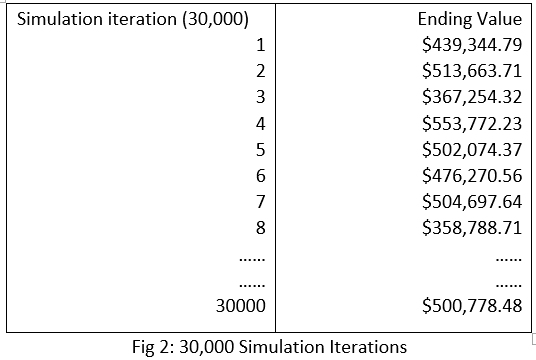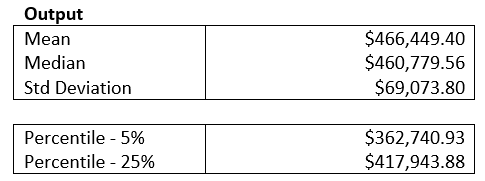# How to Perform Return Analysis with Monte Carlo Simulation# How to Perform Return Analysis with Monte Carlo SimulationIn this article, we share how investors can analyse a fund’s return through a systematic statistical lens, using the simple Monte Carlo simulation method.

The Setup

We performed a Monte Carlo simulation on X investment fund with the following input parameters:In our simulations, we assume an initial investment of \$100,000, with the investment horizon to be 20 years. For simplicity, we assume no further additional investments were made at any time during the horizon, i.e. there are no compound effects on new investments. We further assume X investment fund’s actual average total return (dividends reinvested) was 8% p.a. and the standard deviation was 3.56% on an annualised basis, since the fund’s inceptionFig1 shows a single iteration of a randomly generated scenario based on the inverse of the normal cumulative distribution of X investment fund’s average return and standard deviation. The formula on Excel would look like this:

=NORM.INV(RAND(),\$D\$4,\$D\$5),
where RAND() is Random Probability, \$D\$4 (average return) is 8% and \$D\$5 is 3.56% (standard deviation)

Monte Carlo Simulation

We proceed to perform 30,000 simulations, where each iteration is based on random probabilities but the same parameters.Putting through the 30,000 ending values, we generated the following outputs:The results revealed that the Mean (average) ending value of the investment would return around \$466,500 at the end of the horizon. We further calculated the Median which turned out to be about \$460,800 (no much skewness in data generated). We further computed the 5%- and 25%-percentile of the data distribution arriving at about \$362,700 and \$417,900 respectively.